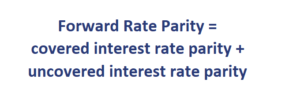# Forward rate parity

Forward rate parity describes the situation in which the forward rate is equal to the future spot rate. In such a situation, the forward rate is an unbiased predictor of the future spot rate. In other words F = E(S1). Under these conditions both the covered interest rate parity and the uncovered interest rate parity hold. While the covered interest rate parity generally holds because of arbitrage activity, the uncovered interest rate parity may not hold in the short run.On this page, we briefly discuss the covered and uncovered interest rate parity. Once we understand both the interest rate parity theorems, it will be easier to grasp forward rate parity. We also discuss the difference between covered and uncovered interest rate parity.

More details on the covered and uncovered interest rate parity are available on another page. On that page, we implement examples of both approaches using an Excel spreadsheet.

## Covered interest rate parity

We briefly discuss the covered interest rate parity. For more information and an Excel example, see covered interest rate parity. Covered interest rate parity refers to a situation where any forward premium or discount in currency forward contracts exactly offsets differences in interest rates. If arbitrage is possible, this condition will generally hold.

## Uncovered interest rate parity

Uncovered interest rate parity is used in situations where arbitrage is not possible or when capital constraints are in place. In such cases, the covered interest rate parity may not hold. If that is the case, can still use the interest rates of the two countries to calculate the expected change in the spot rate. In particular, the interest rate difference can be used to calculate the expected appreciation or depreciation.

The page on the uncovered interest rate parity includes an Excel example that illustrates how to do this.

## Comparing covered and uncovered interest rate parity

Comparing covered and uncovered interest rate parity, we see that the covered version results in the no-arbitrage price of a currency forward. The uncovered interest rate parity provides the expected future spot rate. Thus, while the former is guaranteed by arbitrage, the latter is only an expectation, and may not occur.

If, however, the forward rate is equal to the expected future spot rate, un uncovered interest rate parity holds. This situation is referred to as forward rate parity.

## Summary

On this page we discussed what is covered interest rate parity and uncovered interest rate parity. We noted that the difference between covered and uncovered interest rate parity is the fact that uncovered interest rate parity is not guaranteed by arbitrage.